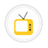you are here->home->Chemical Sciences->Physical Chemistry Virtual Lab
Physical Chemistry Virtual Lab

Physical chemistry (also called physicochemistry) is the explanation of macroscopic, microscopic, atomic, subatomic, and particulate phenomena in chemical systems in terms of physical concepts; sometimes using the principles, practices and concepts of physics like thermodynamics, quantum chemistry, statistical mechanics and dynamics.SpectrophotometryTo apply Beer Lamberts law to solutions and study the effect of concentration on the absorbance of a substanceCryoscopyTo reinforce the concept of colligative properties and to study the effect of solute on the FP substances.EbullioscopyTo reinforce the concept of colligative properties and to study the effect of solute on the BP substances.EMF measurementTo find EMF of the cell. To calculate the Gibbs free energy change of the cell reaction. To calculate the Equilibrium constant. To predict the spontaneity of the cell reaction.Determination of Viscosity of Organic SolventsTo build up the concept of Poiseuilles law and correlate the effect of flow time and viscosity.Adsorption IsothermTo build up the concept of adsorbate,adsorbent,Langumuir and Freundlich Isotherms.Verification of Tafel EquationTo study about the irreversible behavior of an electrode,Understand the mechanism of electron transfer to an electrode and to determine the current density Verifying Tafel plotDetermination of Viscosity Average Molecular Weight of Polymer To build up the concept of Mark-Houwink equation and correlate the effect of flow time and viscosity of a polymer solution.Calorimetry -Water equivalent CalorimetryTo measure heat of reaction or physical changes and heat capacity and to understand the concept of thermodynamics.Calorimetry -Heat of NeutralizationTo measure heat of reaction or physical changes and heat capacity and to understand the concept of thermodynamics.Copyright @ 2023 Under the NME ICT initiative of MHRD